Case Based Questions (MCQ)

Chapter 3 Class 12 Matrices
Serial order wise

## On her birth day, Seema decided to donate some money to children of an orphanage home. If there were 8 children less, everyone would have got Rs. 10 more. However, if there were 16 children more, everyone would have got Rs. 10 less. Let the number of children be x and the amount distributed by Seema for one child be y (in Rs.).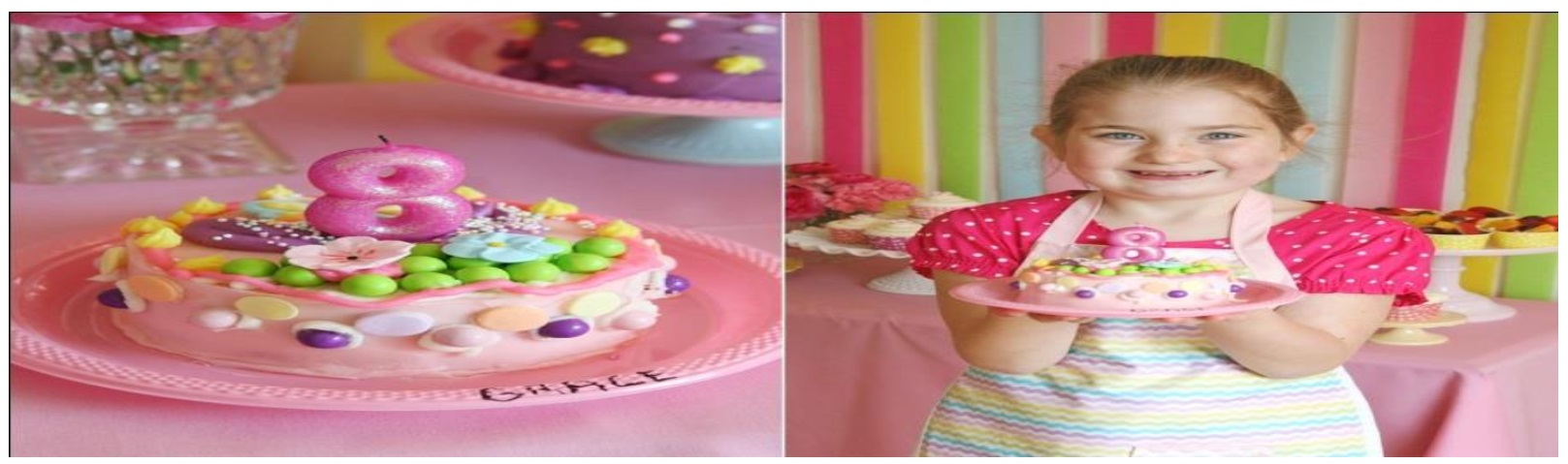googletag.cmd.push(function() { googletag.display('div-gpt-ad-1669298377854-0'); }); Based on the information given above, answer the following questions: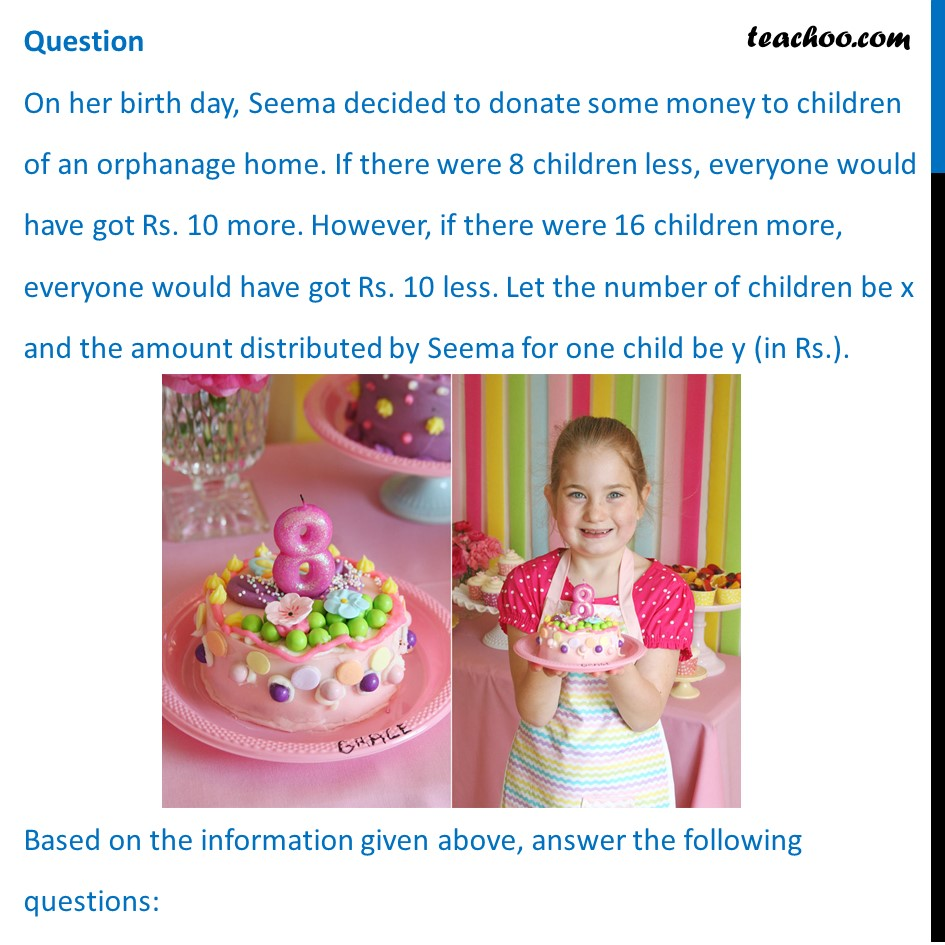## (a) 5x – 4y = 40       5x – 8y = –80 (b) 5x – 4y = 40       5x – 8y = 80 (c) 5x – 4y = 40       5x + 8 y = –80 (d) 5x + 4y = 40       5x – 8y = –80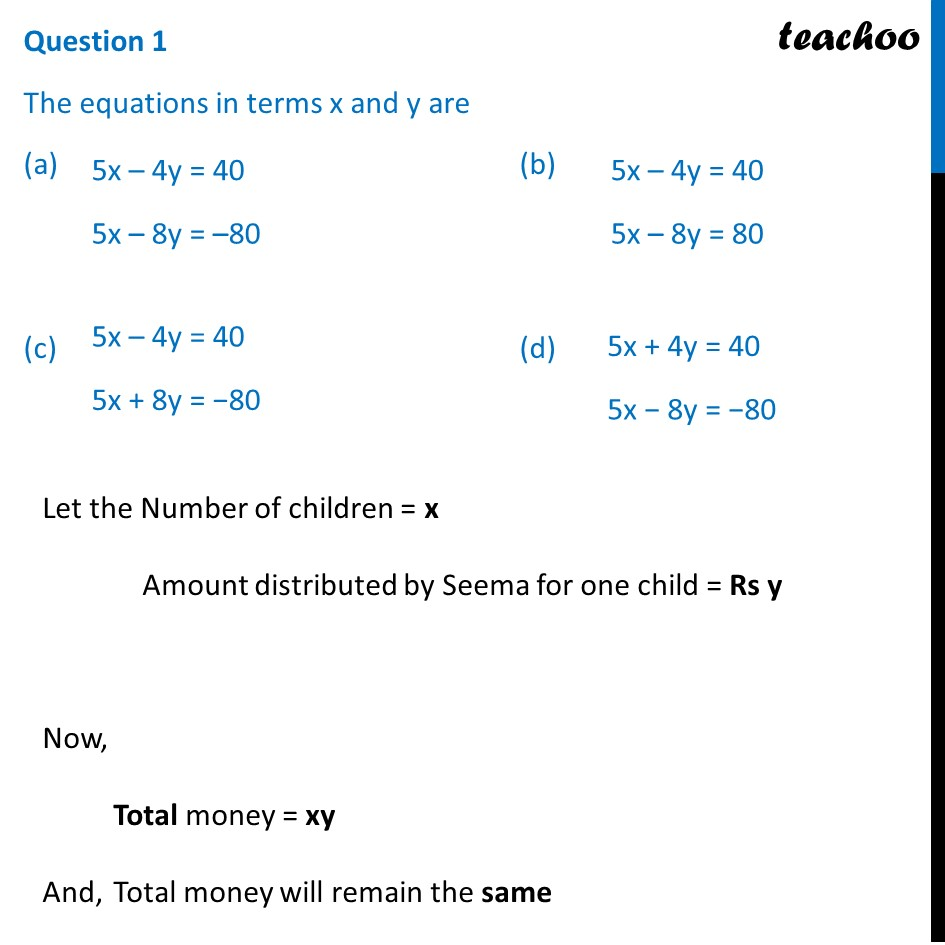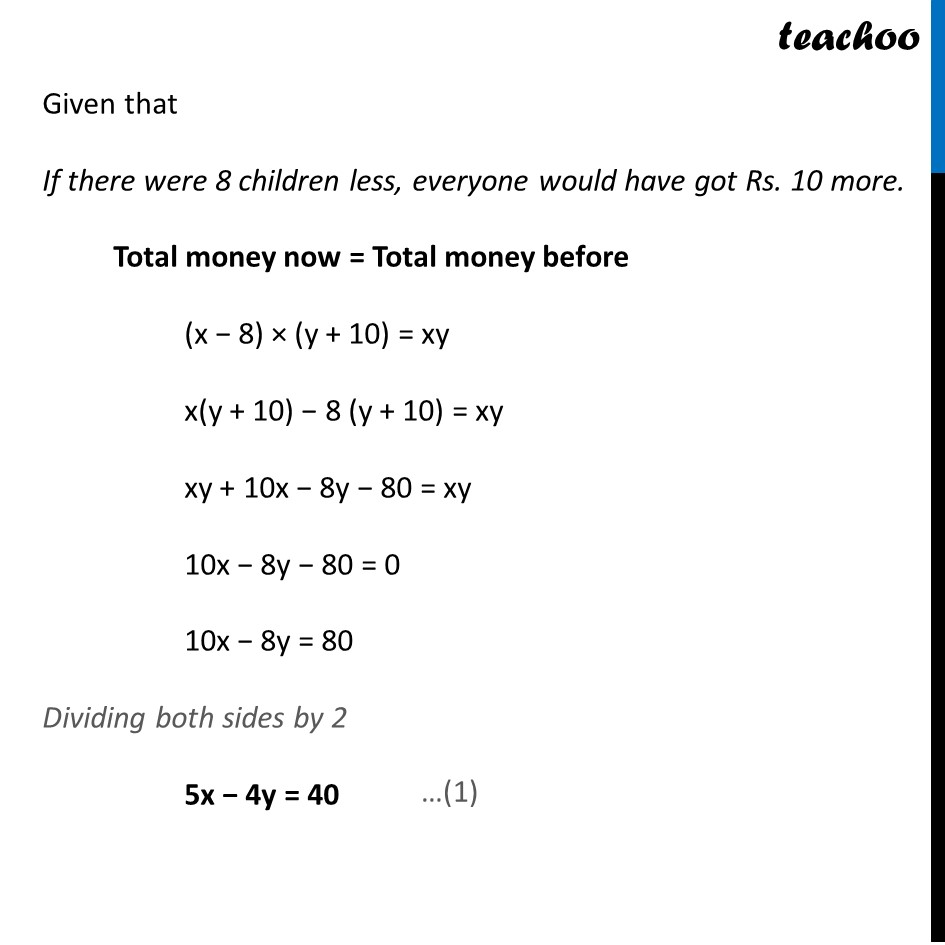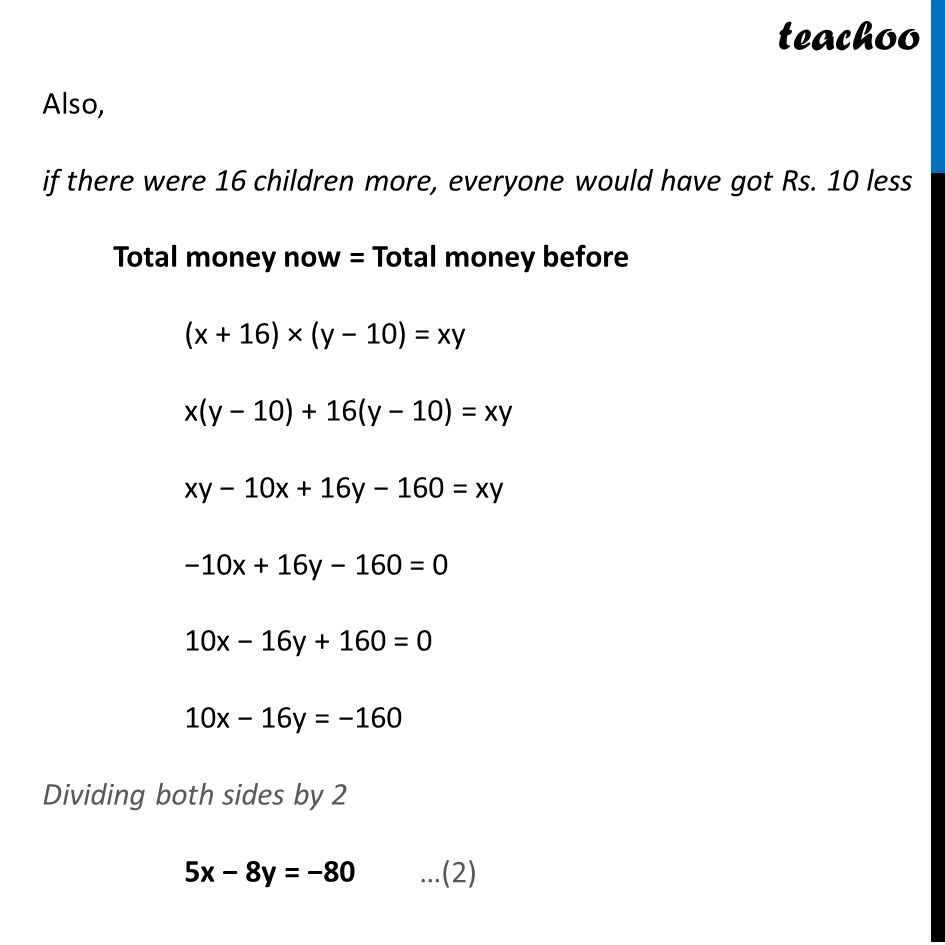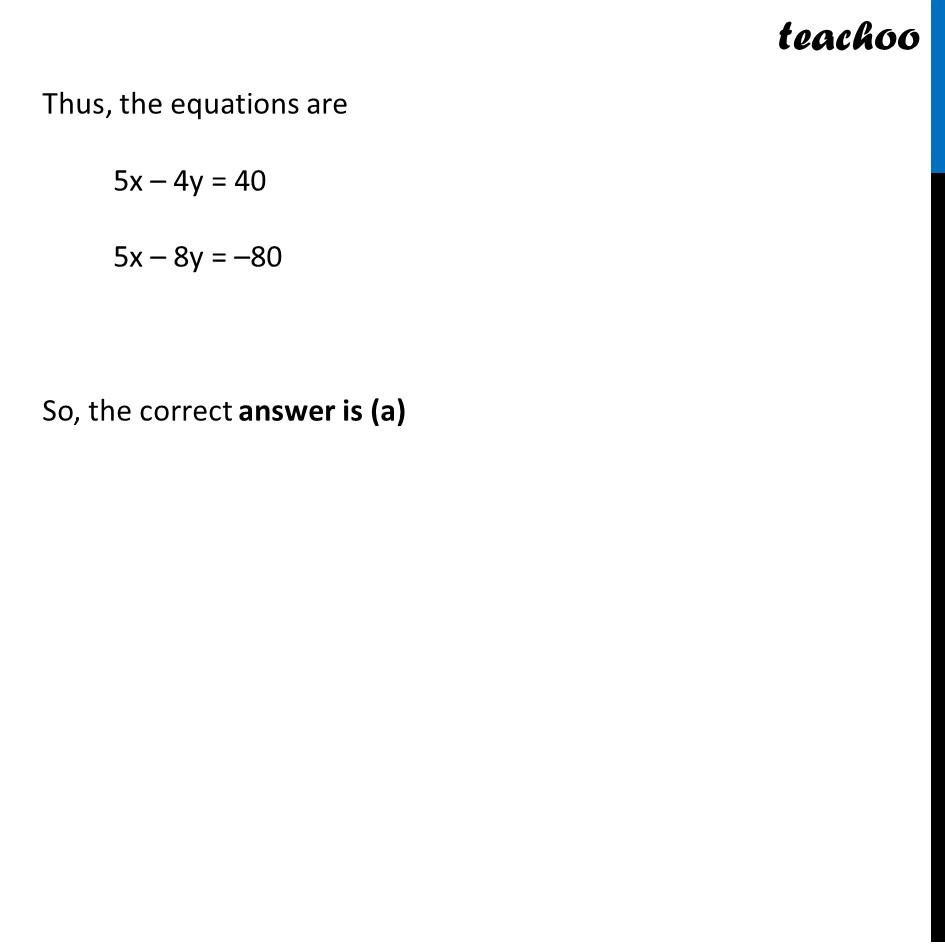## (d) [5  4    [x / y]   = [40 / -80]       5   -8]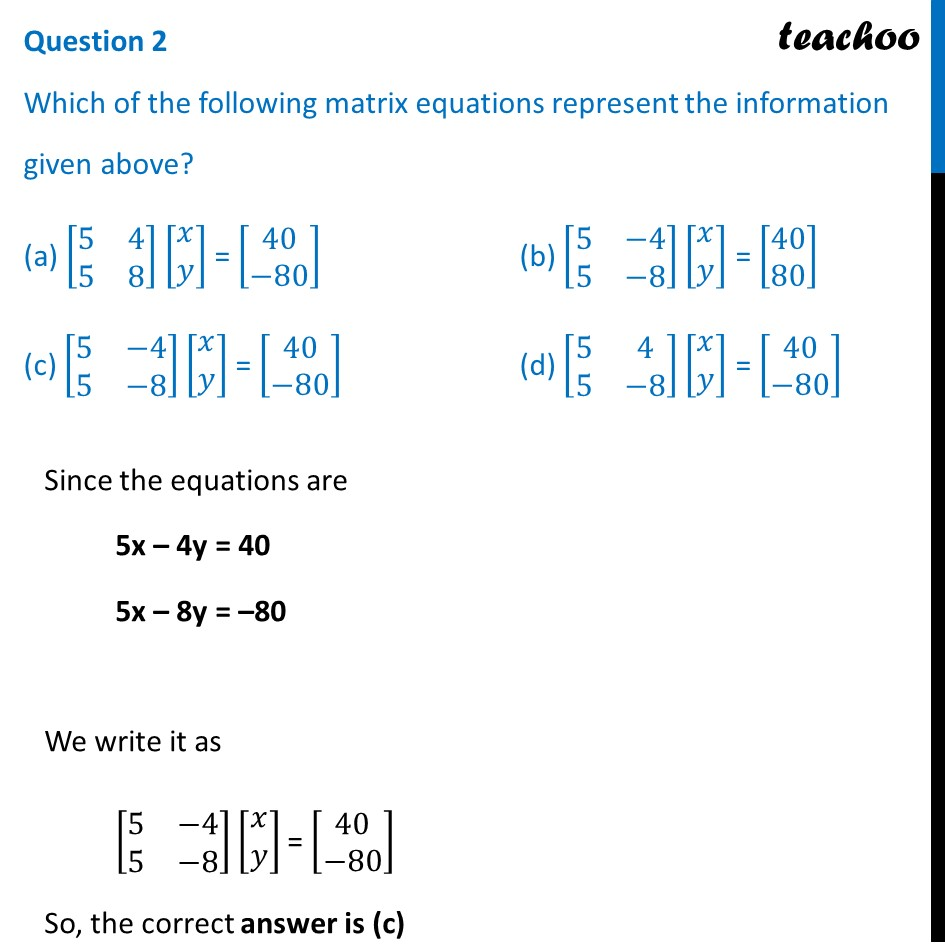## (a) 30 (b) 40 (c) 23 (d) 32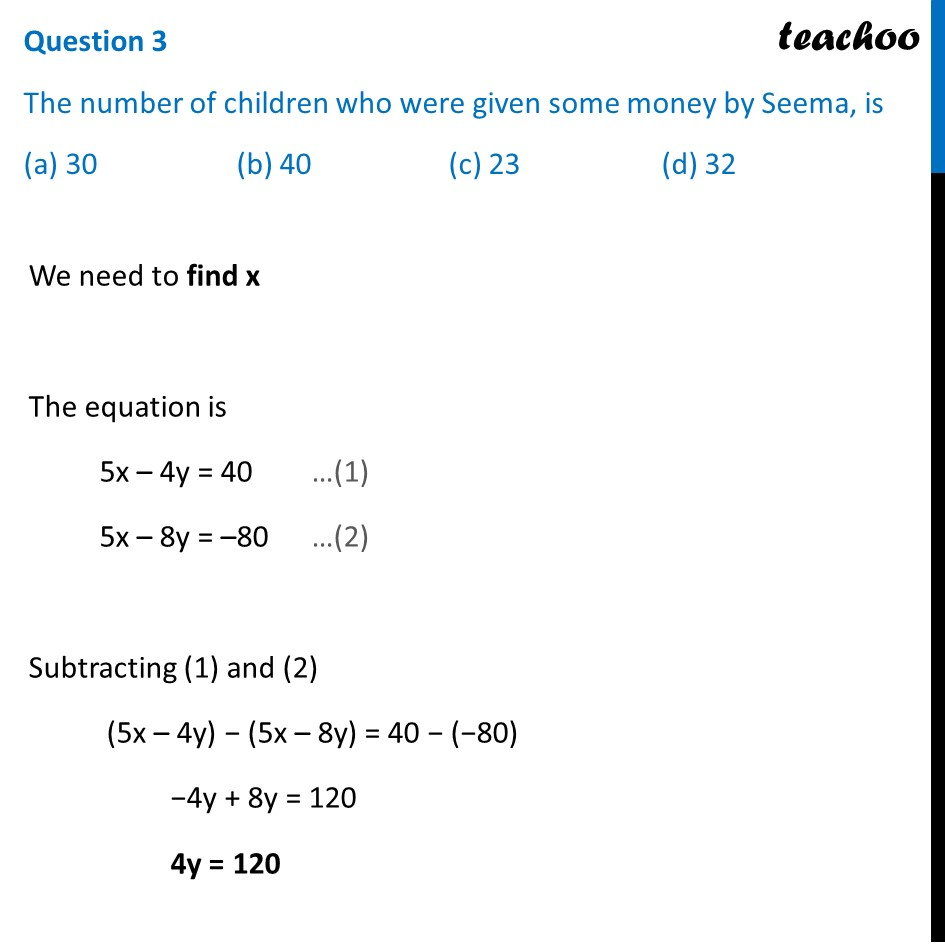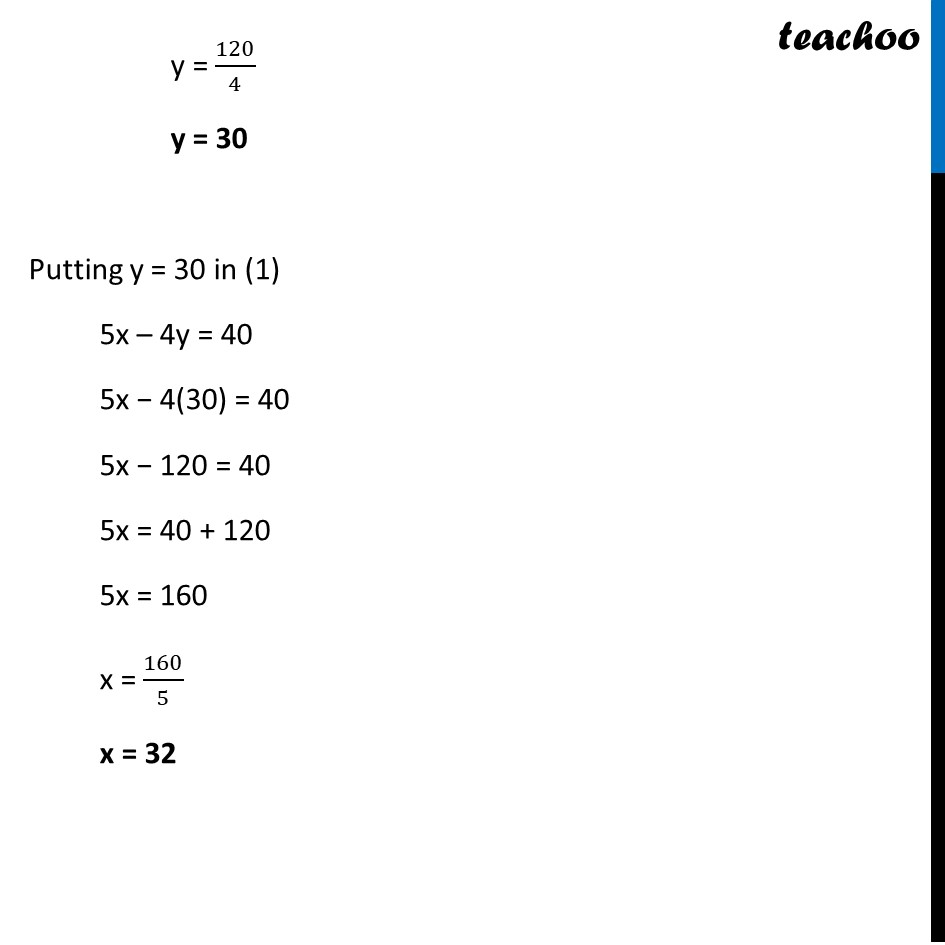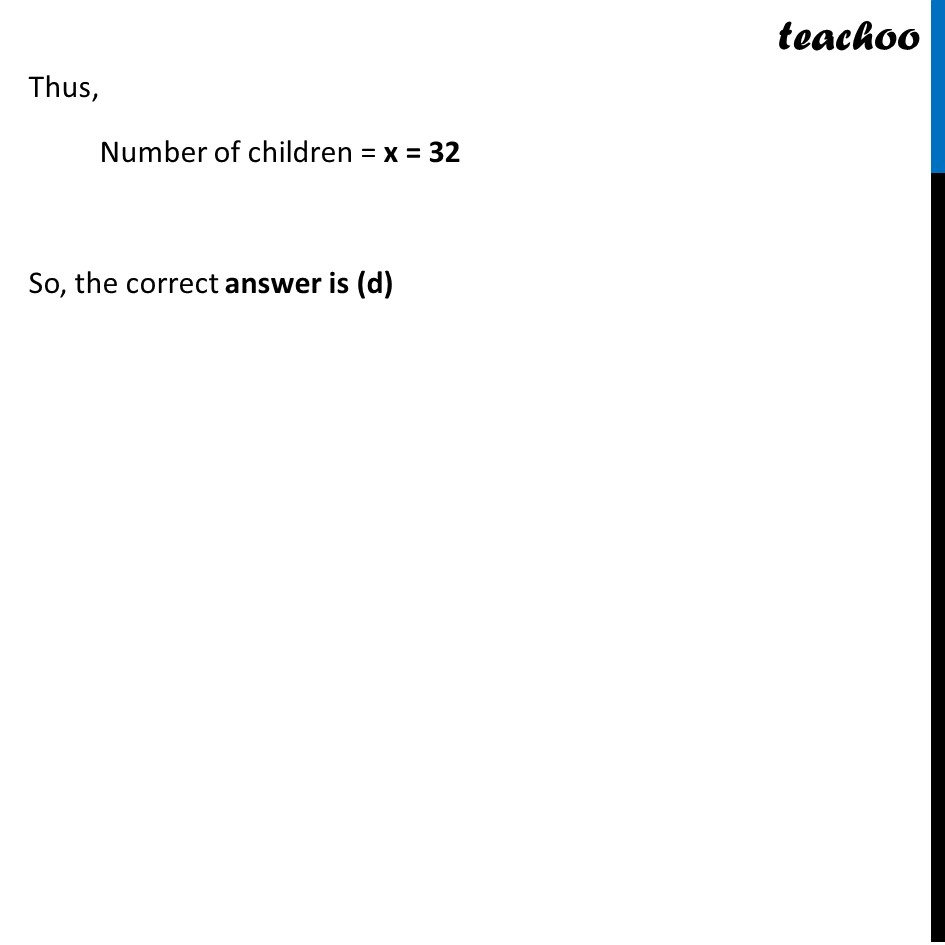## (a) Rs. 32 (b) Rs. 30 (c) Rs. 62 (d) Rs. 26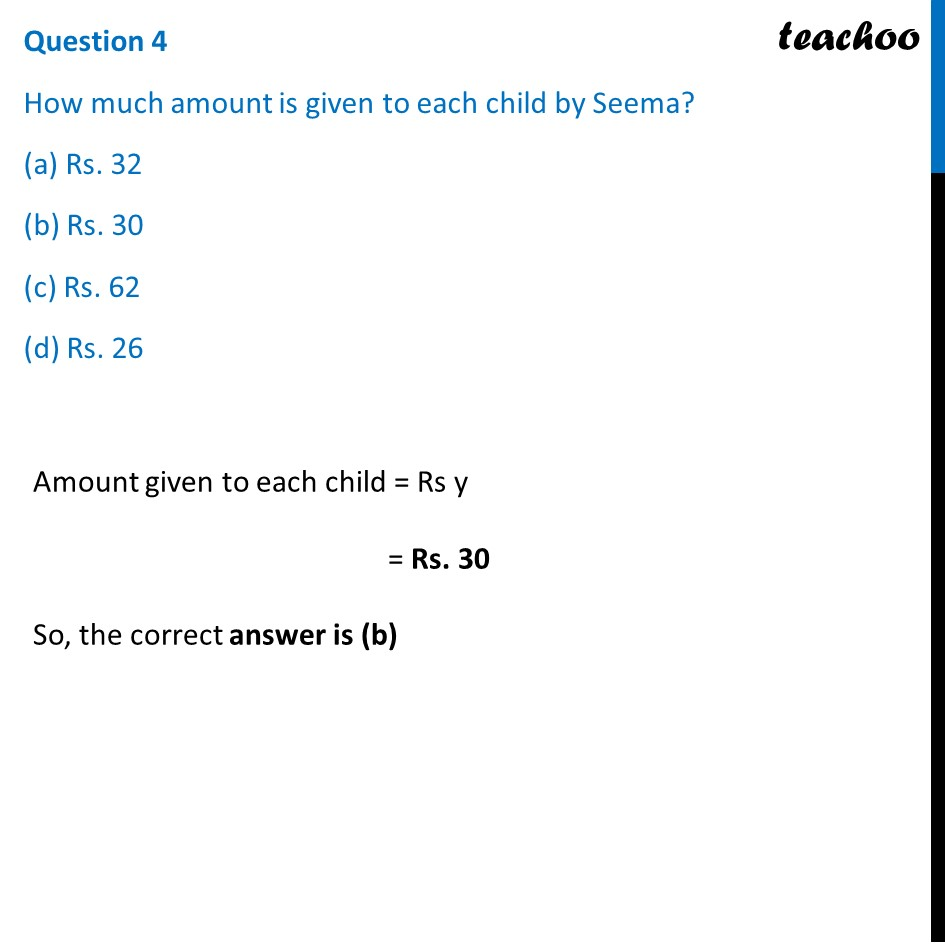## (a) Rs. 609 (b) Rs. 960 (c) Rs. 906 (d) Rs. 690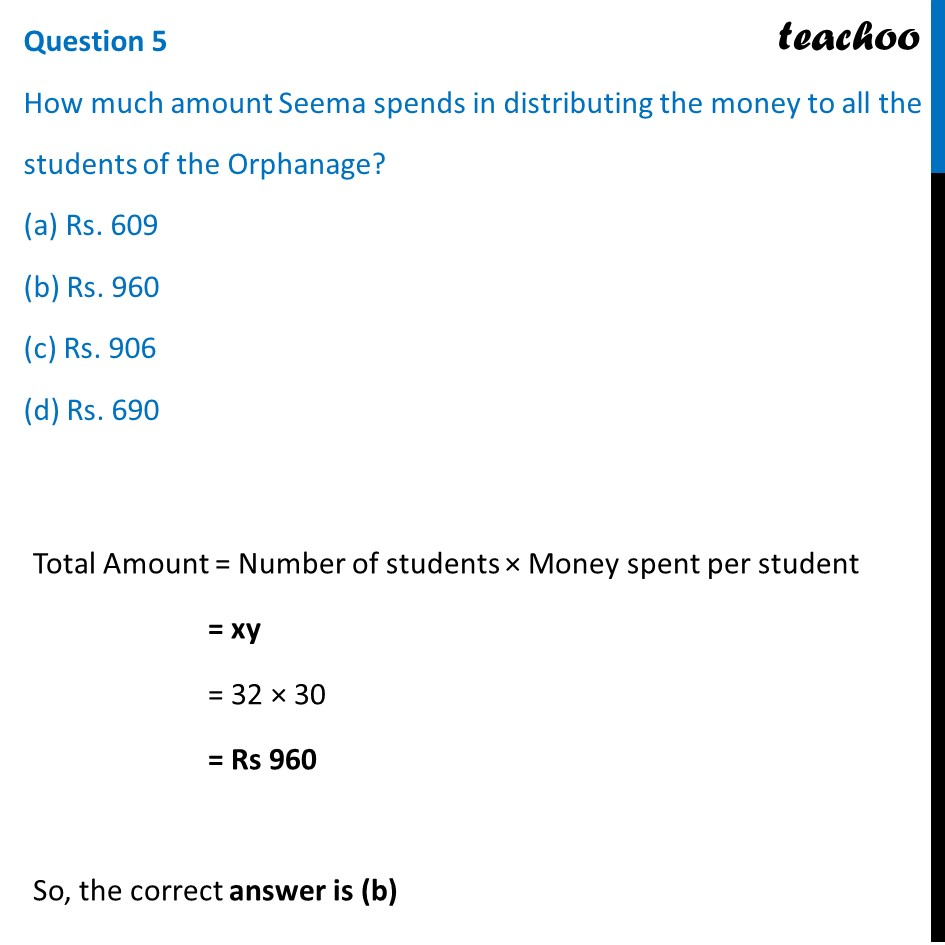Learn in your speed, with individual attention - Teachoo Maths 1-on-1 Class

### Transcript

Question On her birth day, Seema decided to donate some money to children of an orphanage home. If there were 8 children less, everyone would have got Rs. 10 more. However, if there were 16 children more, everyone would have got Rs. 10 less. Let the number of children be x and the amount distributed by Seema for one child be y (in Rs.). Based on the information given above, answer the following questions:Question 1 The equations in terms x and y are (a) 5x – 4y = 40 5x – 8y = –80 (b) 5x – 4y = 40 5x – 8y = 80 (c) 5x – 4y = 40 5x + 8 y = –80 (d) 5x + 4y = 40 5x – 8y = –80(a) 5x – 4y = 40 5x – 8y = –80 Let the Number of children = x Amount distributed by Seema for one child = Rs y Now, Total money = xy Given that If there were 8 children less, everyone would have got Rs. 10 more. Total money now = Total money before (x − 8) × (y + 10) = xy x(y + 10) − 8 (y + 10) = xy xy + 10x − 8y − 80 = xy 10x − 8y − 80 = 0 10x − 8y = 80 Dividing both sides by 2 5x − 4y = 40 Also, if there were 16 children more, everyone would have got Rs. 10 less Total money now = Total money before (x + 16) × (y − 10) = xy x(y − 10) + 16(y − 10) = xy xy − 10x + 16y − 160 = xy −10x + 16y − 160 = 0 10x − 16y + 160 = 0 10x − 16y = −160 Dividing both sides by 2 5x − 8y = −80 Thus, the equations are 5x – 4y = 40 5x – 8y = –80 So, the correct answer is (a) Question 2 Which of the following matrix equations represent the information given above? (a) [■8(5&4@5&8)] [■8(𝑥@𝑦)] = [■8(40@−80)] (b) [■8(5&−4@5&−8)] [■8(𝑥@𝑦)] = [■8(40@80)] (c) [■8(5&−4@5&−8)] [■8(𝑥@𝑦)] = [■8(40@−80)] (d) [■8(5&4@5&−8)] [■8(𝑥@𝑦)] = [■8(40@−80)] Since the equations are 5x – 4y = 40 5x – 8y = –80 We write it as [■8(5&−4@5&−8)] [■8(𝑥@𝑦)] = [■8(40@−80)] So, the correct answer is (c) Question 3 The number of children who were given some money by Seema, is (a) 30 (b) 40 (c) 23 (d) 32 We need to find x The equation is 5x – 4y = 40 …(1) 5x – 8y = –80 …(2) Subtracting (1) and (2) (5x – 4y) − (5x – 8y) = 40 − (−80) −4y + 8y = 120 4y = 120 So, the correct answer is (a) y = 120/4 y = 30 Putting y = 30 in (1) 5x – 4y = 40 5x − 4(30) = 40 5x − 120 = 40 5x = 40 + 120 5x = 160 x = 160/5 x = 32 Thus, Number of children = x = 32 So, the correct answer is (d) Question 4 How much amount is given to each child by Seema? (a) Rs. 32 (b) Rs. 30 (c) Rs. 62 (d) Rs. 26 Amount given to each child = Rs y = Rs. 30 So, the correct answer is (b) Question 5 How much amount Seema spends in distributing the money to all the students of the Orphanage? (a) Rs. 609 (b) Rs. 960 (c) Rs. 906 (d) Rs. 690 Total Amount = Number of students × Money spent per student = xy = 32 × 30 = Rs 960 So, the correct answer is (b)